# Main goal of the article :

The main goal of the article is to demonstrate a project that makes use of a training dataset containing labeled face and non-face images to train an Adaboost classifier that classifies whether a given image is a face or a non-face and use test images to test the accuracy of the learned classifier.

# Programming language and packages we have used:

We have used Python 3.6 as our programming language and the following python packages:

• Pickle
• Numpy
• Scikit-learn
• Matplotlib
• Tqdm
• Pandas
• Pillow

# Main activities performed sequentially in the project:

The initial task was to find proper datasets containing face and non-face images for our project.

For the positive class (face images), we have used the dataset A Century of Portraits: A Visual Historical Record of American High School Yearbooks. It contains 37,921 pictures of frontal-facing portraits out of which we have used 2000 face-images due to restraints in computing resources.

For the negative class (non-face images) we have used the Stanford background dataset from http://dags.stanford.edu/projects/scenedataset.html containing 715 images of various backgrounds which are not “faces”. To balance our dataset, we have used data augmentation to increase our dataset size to 1430 images by randomly cropping regions from the original 715 images which we have used as non-face images.

We have re-sized all of our images to a uniform size of 22x22 and split our entire dataset into a training set containing 1600 face and 1144 non-face images and a test set containing 400 face and 286 non-face images.

• Grayscale Conversion:

Color information of the images was of no use to us in Face detection. Therefore, we have converted the images to grayscale images.

Face images after grayscale conversion Non-Face images after grayscale conversion

• Computing Integral Image:

Rectangular features can be computed rapidly using Integral images. An integral image at location (x,y) is calculated using the sum of the pixels above and to the left of x, y, inclusive.

The image below depicts the calculation for an integral image.

Using the Integral image, any rectangular sum can be computed using four array references. Since the process of extracting haar-like features involves computation of the rectangular sum of lighter/darker regions, the introduction of Integral images greatly speeds up the process. We have computed integral images for our entire dataset. We then proceeded to build our Haar-like features.

• Extracting Haar Features:

Viola and Jones in their paper has defined the following 3 types of Haar-like features as follows:-

• Edge features
• Line feature
• Four-sided feature

In addition to the above four features, we have considered a fifth feature of type Line feature in our project as follows:-

• Fifth line feature

In the code, we have created a dictionary for the above five types of Haar-like features which stores tuples denoting our individual Haar features:-

The total number of features of different types for images of size 22x22 are calculated as shown below:

When these features are overlaid on the image, values corresponding to light regions are added and the values corresponding to dark regions are subtracted from the above sum. These haar-like features help us to extract useful information such as edges, straight lines, and diagonals that can be used to identify objects, e.g. a human face.

Even for small images, we would get a lot of haar features, in our case over 110,000 features for a 22 x 22 image. To compute all the features efficiently Viola and Jones introduced the integral image which we have mentioned in the previous step.

Using Integral images the sum of the pixels within rectangle D in the above image can be computed with four array references. Sum of the pixels in rectangle A gives the value of the integral image at location 1 in the above image. A+B gives the value at location 2, A+C gives the value at location 3 is , and A+B+C+D gives the value at location 4. The sum within D can be computed as 4+1 — (2+3). Using this method we can calculate the values of all the Haar-like features.

• Viola-Jones Algorithm:

Viola-Jones algorithm had used a variant of AdaBoost for training. We have used the following steps in our algorithm:

1. Initialized weights for each training example
2. Normalized all the weights
3. Then we selected the best weak classifier based on the weighted error of the training examples
4. Then updated the weights according to the error of the chosen best weak classifier
5. Repeated steps 2–4 N times, where N is the desired number of weak classifiers

A detailed description of the steps implemented are as follows:

1. Initialized weights for each training example

At the start of the algorithm, we gave each training example the same weight. If the positive classes are p in number and negative classes are n in number, then we have assigned the following weights to the training examples:

Where x is the class of the training example.

2. Building the features

The training function requires choosing the best weak classifier and each possible feature gives one weak classifier. So we have built all of the features before implementing the main loop of training and stored the type of each feature (from the 5 Haar-like feature types defined). The algorithm loops over all rectangles in the image and checks if it is possible to make a feature with it.

3. Applying the features

We have applied the features before we have started training the classifiers because the value of each feature for the images never changes. Applying the features before training also allowed us to pre-select features (we have used SelectPercentile from sklearn.feature_selection library to pre-select features) later on to speed up training.

4. Training the Weak Classifiers

Viola-Jones uses a series of weak classifiers and combines them according to their weights to obtain a final strong classifier. Each weak classifier looks at a single feature( f ). Each weak classifier has a threshold ( θ ) and a polarity ( p ) to determine the classification of a training example according to the following equation:

Each feature is a sum of the positive rectangle regions with the sum of the negative rectangle regions subtracted from them.

For each feature, we have trained a single classifier to find its optimal threshold and polarity using the weights of the training examples. Each time a new weak classifier is selected as the best one, all of the weak classifiers have to be retrained since the training examples are weighted differently after each round. Since this is a computationally expensive process we have used an optimized method to do the training of a weak classifier. We have first sorted the weights according to the feature value that they correspond to. Then we iterated through the array of weights and computed the error if the threshold was chosen to be that feature. The error was computed using the following equation:

error=minimum( P+TN-N , N+TP-P )

where,

P=sum of weights of all the positive examples seen so far

N=sum of weights of all the negative examples seen so far

TP=Total sum of weights of all the positive examples

TN=Total sum of weights of all the negative examples

Using this equation we can find the error of each threshold in constant time and the error of all the thresholds in linear time. We find the threshold and polarity with the minimum error. The possible values for a threshold are the values of the feature on each training example. The threshold is set to the value of the feature at which the error is minimum. The polarity is determined by how many positive examples and negative examples have feature values (from the particular feature under consideration) lesser or and greater than the threshold.

Polarity p=1, If there are more positive examples with feature values less than the threshold, else p=-1.

5. Selecting the best weak classifier

To select the best weak classifier in each round, we iterated through all the classifiers and calculated the average weighted error of each one on the training dataset, and chose the classifier with the lowest error.

6. Updating the Weights

After choosing the best weak classifier, we update the weights with the error of the chosen weak classifier. Training examples that were classified correctly are assigned smaller weights, examples which were classified incorrectly have no change in their weights. We are updating the weights according to the following equations:

Wi is the weight of the ith example, 𝜖 is given by the error of the best weak classifier, and 𝜷 which is obtained using the equation above, gives the factor by which we change the weights. The exponent of 𝜷 is 1-e where e is 0 if the training example was classified correctly and 1 if classified incorrectly.

7. The Strong Classifier

The final classifier is defined as:

The final classifier is a weighted linear combination of T (in our case T=10) number of weak classifiers where the weights (denoted by coefficient 𝛂) are dependent on the error since it is the natural log of the inverse of 𝜷. If the weighted sum of the weak classifiers’ decisions is greater than or equal to half the sum of all the 𝛂 then we assign it to class 1 else it is assigned to class 0.

The attentional cascade uses a series of Viola-Jones classifiers, each progressively more complex, to classify an image. The classifiers are used in a cascade fashion in which only the first classifier trains on all the training examples, and each subsequent classifier is trained on all positive examples and the negative examples that the previous classifier misclassified i.e, the false positives.

# Main Results of the project:

We ran our algorithm for 10 rounds. Following are the details of the weak classifiers selected by Adaboost in each round:

So these are the 10 features selected by Adaboost in 10 rounds as shown below:-

Haar feature: Vertical_2_rectangle, Feature details: (1, 2), x=13, y=7, width=3, height=2

Haar feature: Horizontal_2_rectangle, Feature details: (2, 1), x=13, y=0, width=6, height=2

Haar feature: Horizontal_2_rectangle, Feature details: (2, 1), x=10, y=5, width=4, height=2

Haar feature: Vertical_2_rectangle, Feature details: (1, 2), x=1, y=0, width=1, height=22

Haar feature: Vertical_2_rectangle, Feature details: (1, 2), x=5, y=4, width=5, height=2

Haar feature: Vertical_2_rectangle, Feature details: (1, 2), x=1, y=1, width=7, height=14

Haar feature: Vertical_3_rectangle, Feature details: (3, 1), x=9, y=20, width=3, height=2

Haar feature: Vertical_2_rectangle, Feature details: (1, 2), x=3, y=6, width=2, height=4

Haar feature: Horizontal_2_rectangle, Feature details: (2, 1), x=10, y=2, width=10, height=1

Haar feature: Vertical_2_rectangle, Feature details: (1, 2), x=15, y=0, width=1, height=16

Images below show the top feature in every round:

From the above pictures, we can see that Adaboost features are somewhat trying to capture the following details from the face:

1. Eye details (Round 5)
2. Lip details (Round 10 and somewhat in Round 1)
3. Eyebrow details (Round 8)
4. Ear details (Round 9, Round 7)
6. Probably hair and ear details (Round 2)
7. Round 6 is trying to capture some details regarding eyes and nose together.

Evaluation metrics of the classifier on our training dataset:

Accuracy obtained on the training dataset after 10 rounds is 97.9227%. Maximum accuracy obtained is in round 9 with a value of 98.3965%.

Following are the evaluation metrics details:

Evaluation metrics of the classifier on our testing dataset:

Accuracy obtained on the testing dataset after 10 rounds is 96.7930%. Maximum accuracy obtained is in round 8 with a value of 97.3761%.

Following are the evaluation metrics details:

ROC Curve of the combined classifier after running 1, 2, 3, 4, 5, 6, 7, 8, 9, and 10 boosting rounds and applied on the test dataset:

A receiver operating characteristic curve, or ROC curve, is a graphical plot that illustrates the diagnostic ability of a binary classifier system as its discrimination threshold is varied.

From the above equation, we can see that the threshold of the combined classifier is given by ½ * sum(𝛂). We varied this threshold of the combined classifier to calculate the False Positive Rate and True Positive Rate for each of the thresholds chosen, which we then plotted to get the ROC curve below.

From the ROC curve we can make the following observations:

• The classifier in each round is a good classifier because of the following reasons:
• The classifier ROC curve has a greater Area under the curve (AUC) than the line y=x which is the line passing through the diagonal of the graph. The diagonal y=x line represents a random classifier that randomly classifies images as faces and non-faces without any ability to distinguish between the 2 classes. We know that the better the classifier, the greater its AUC will be. So our classifier performs better than a random classifier.
• Since the ROC curves of the classifier are far away from the diagonal line, hence we can say that we have a good classifier
• We are obtaining a high True positive rate (TPR) at a low False positive rate (FPR) for our classifier indicating that we have a good classifier.
• As boosting rounds increases, our classifier is getting better because of the following reasons:
• The AUC of the ROC curves increases with an increase in boosting rounds indicating that we have a better classifier
• We are obtaining a higher True positive rate (TPR) at a lower False positive rate (FPR) with an increase in boosting rounds indicating that we have a better classifier
• The ROC curves are getting farther away from the diagonal line with each boosting rounds
• From the graph, we can observe that the ROC curves for Rounds 6, 7, 8, 9, 10 are almost indistinguishable from each other indicating that there is not much enhancement in the performance of the classifier after a certain number of rounds. This observation can help us figure out the optimal number of boosting rounds required to train the classifier.

# Challenges Faced and Steps taken to overcome them:

A. We were not able to find proper labelled datasets containing both faces and non-faces images together for training and testing purposes.

Steps taken to overcome:

We have used face images from one source and non-face images from a different source and manually combined and labelled them together to create out training and testing datasets.

B. We had restraints on the computing resources to train our model.

Steps taken in order to avoid that:

1. We have re-sized all of our images to a uniform size of 22x22.
2. Our face images data source contained 37,921 pictures of frontal-facing portraits out of which we have used 2000 face images.
3. We used Google Colab to run our entire code which provided us with better computational resources

C. Our non-face images data source contained only 715 images which was causing an imbalance of positive and negative class images in our dataset.

Steps taken to overcome:

To balance our dataset, we have used data-augmentation to increase our non-face images dataset size to 1430 images by randomly cropping regions from the original 715 images.

D. Even for small images, we would get a lot of haar features, in our case over 110,000 features for a 22 x 22 image. Each Adaboost round was taking a lot of time to complete. Moreover, many of these features didn’t offer much classification power and were mostly useless.

Steps taken to overcome:

We have used the SelectPercentile class in the SciKit-Learn package which selects features according to a percentile of the highest scores, in order to narrow down our feature space. Using this we have chosen the top 10% of the potential features. With this, each Adaboost round was taking a lot less time to complete.

# References:

 P. Viola and M. Jones, “Rapid object detection using a boosted cascade of simple features,” Proceedings of the 2001 IEEE Computer Society Conference on Computer Vision and Pattern Recognition. CVPR 2001, 2001, pp. I-I, doi: 10.1109/CVPR.2001.990517.

--

--

--

Love podcasts or audiobooks? Learn on the go with our new app.

## En-Lightning Reinforcement Learning## Deep Learning: Style Transfer## Kubeflow 0.3 Simplifies Setup & Improves ML Development## Building Pinterest Lens: a real world visual discovery system## Soft Sign Activation Function with Tensorflow [ Manual Back Prop with TF ]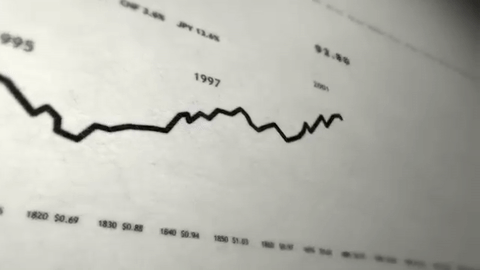## To be(rt) or not to be(rt) 🤗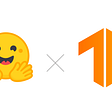## ML & DL —Deep Artificial Neural Networks (Part 5)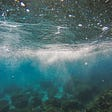## User Identification in Pinterest Through the Refinement of Cascade Fusion of Text and Images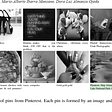## Handwritten Character Recognition Web App with EMNIST## MiniMax Algorithm Explanation using Tic-Tac-Toe Game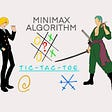## The Basic Classification of Thyroid Tumors on UltraSound Images using Deep Learning Methods## Instantaneous Camera Meal Tracking with Macro